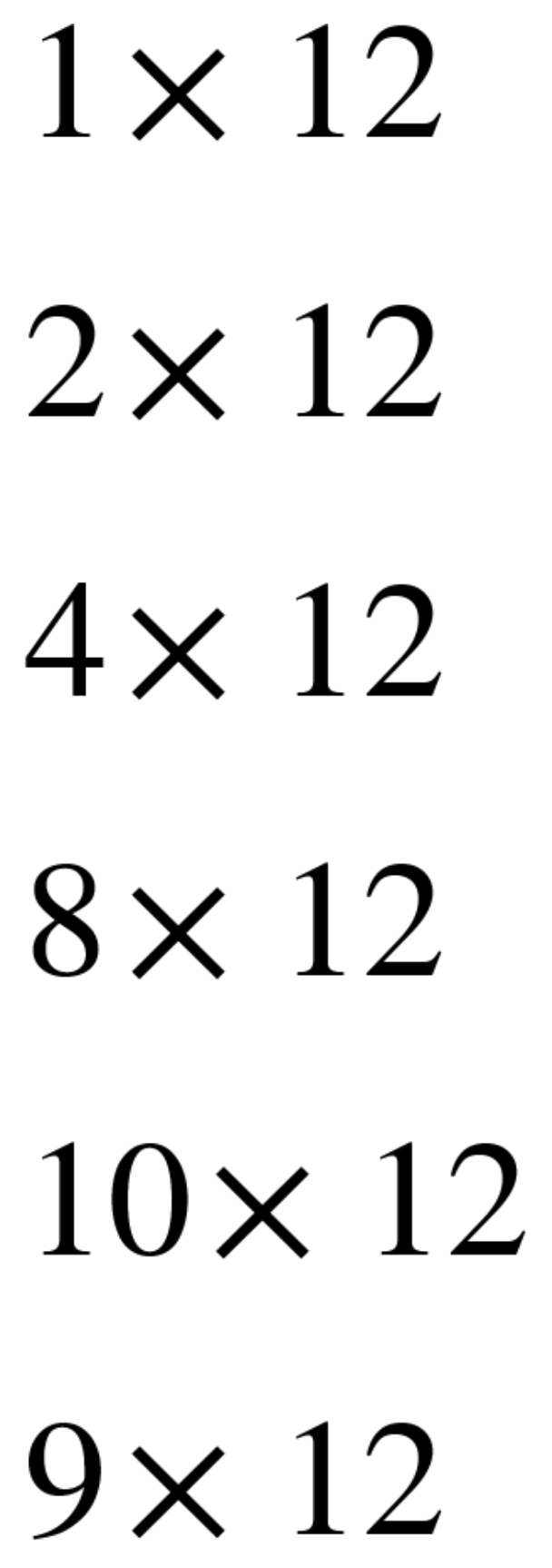< Back to Building the Ratio Table: 12 Sticks of Gum

## About the Mathematics & StructureThis Problem String has 3 problems that have the potential to suggest doubling as a strategy, 2 × 12, 4 × 12, 8 × 12.

Then the fourth and fifth problems, 10 × 12, 9 × 12 are related by one pack of gum. The 10 × 12 can be created by two nice strategies, using 2 × 12 and 8 × 12 or by using the 'times 10' pattern in our number system. Similarly the next problem, 9 × 12 can be found by using 1 × 12 and 8 × 12 or 10 × 12 and 1 × 12.

The number of sticks in a pack, 12, is purposefully chosen because it is easy to double but also because then that number, 24, is also relatively easy to double. It is not until 8 packs that 48 will take some deeper thinking for students to double. It is also a relatively easy number of sticks to subtract from 120 sticks in 10 packs to find the number of sticks in nine packs. If we had used a smaller number of sticks, we would run more of a risk that some students would "just know" all of the multiples of that number, so that would limit the thinking that would happen. If we use a number of sticks that is higher, the numbers become harder to double and subtract from 10 packs.

To Facilitate the String:

As you prepare to lead your class through this Problem String, consider the following sample language, strategies to pull out from students, and ideas to highlight:

• If there are 12 sticks in one pack of gum, how many sticks are in 2 packs? If you double the number of packs, you double the number of sticks? How? Represent scaling in tandem.
• Repeat for each question.
• For 10 packs, ask for 8 + 2 before times 10.
• For 9 packs, ask for 8 + 1 before 10 − 1.
• Which of those two strategies, the 8 + 1 or the 10 − 1, makes use of a problem one usually already knows? Which is more efficient?

Important Questions:

Consider what you could ask at key times in the Problem String to help students build models, strategies, and big ideas:

• How could you use other entries in the table to help you?
• Which of those strategies is more efficient, if you do not have the rest of the information in the table? If you only know how many sticks are in one pack?